COS 126 Hamming Codes in TOY Programming Assignment Due: Thursday, 11:59pm

Write a TOY program to encode data using Hamming codes. Then write a TOY program to correct encoded data that has been corrupted.

Perspective.  Error correcting codes enable data to be sent through a noisy communication channel without corruption. To accomplish this, the sender appends redundant information to the message, so that even if some of the original data is corrupted during transmission, the receiver can still recover the original message intact. Transmission errors are common and can arise from scratches on a CD, static on a cell phone, or atmospheric interference. In a noisy environment, error correcting codes can increase the throughput of a communication link since there is no need to retransmit the message if it becomes partially corrupted during transmission. For this reason, error correcting codes are used in many common systems including: storage devices (CD, DVD, DRAM), mobile communication (cellular telephones, wireless, microwave links), digital television, and high-speed modems (ADSL, xDSL).

Hamming Codes.   A Hamming code is a specific type of error correcting code that allows the detection and correction of single bit transmission errors. Hamming codes are used in many applications where such errors are common, including DRAM memory chips and satellite communication hardware. Hamming codes work by repeatedly reading four message bits, which we denote by m1, m2, m3, m4, and then inserting three parity bits, which we denote by p1, p2, and p3. If any one of these seven bits is corrupted during transmission, the receiver can detect the error and recover the original four message bits intact. This is called single bit error correction because at most one bit can be corrected per unit of data sent. The overhead for using this method is a 1.75 times increase in the amount of bandwidth since we use three extra parity bits for every four message bits. This compares favorably with naive approaches, e.g., sending three copies of each bit and using the one that appears most frequently.

Before we describe the algebra of Hamming codes, we first visualize the calculation of these parity bits using Venn diagrams. As an example, suppose we wish to send the message 1101. We associate each of the four message bits with a specific intersection region of three pairwise overlapping circles, as illustrated below: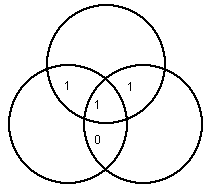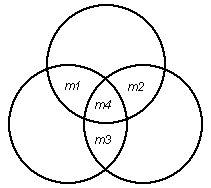The Hamming code adds three parity bits so that each circle has even parity.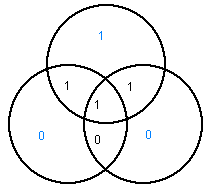That is, the sum of the four bits in each circle is now even: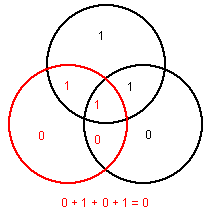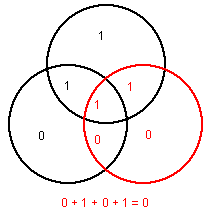In this case we send 1101100 since the three parity bits are 1 (top), 0 (left), and 0 (right).

Now imagine this picture is transmitted via modem over a noisy communiation channel, and that one bit is corrupted so that the following picture arrives at the receiving station (corresponding to 1001100: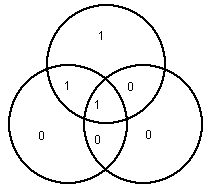The receiver discovers that an error occurred by checking the parity of the three circles. Moreover, the receiver can even determine where the error occurred (the second bit) and recover the four original message bits!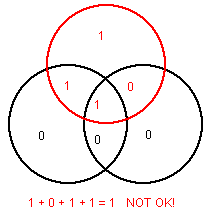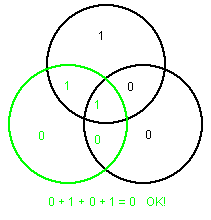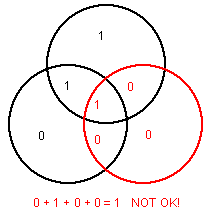Since the parity check for the top circle and right circle failed, but the left circle was ok, there is only one bit that could be responsible, and that's m2. If the center bit, m4, is corrupted then all three parity checks will fail. If a parity bit itself is corrupted, then only one parity check will fail. If the data link is so noisy that two or more bits are simultaneously corrupted, then our scheme will not work. Can you see why? More sophisticated types of error correcting codes can and do handle such situations.

Of course, in practice, only the 7 bits are transmitted, rather than the circle diagrams.

Part 1. Write a TOY program encode.toy to encode a binary message using the scheme described above. Your program should repeatedly read four bits m1, m2, m3, and m4 from TOY standard input, and output the seven bits m1, m2, m3, m4, p1, p2, p3 to TOY standard output, where

• p1 = m1 ^ m2 ^ m4
• p2 = m1 ^ m3 ^ m4
• p3 = m2 ^ m3 ^ m4
• Recall that ^ is the XOR operator in Java and TOY. This captures the parity notion described above.

Part 2. Write a TOY program decode.toy to correct a Hamming encoded message. Your program should repeatedly read seven bits m1, m2, m3, m4, p1, p2, p3 from TOY standard input, and output four bits to TOY standard output. Recall, to determine which one, if any, of the message bits is corrupted, perform the following three parity checks:

1. p1 = m1 ^ m2 ^ m4
2. p2 = m1 ^ m3 ^ m4
3. p3 = m2 ^ m3 ^ m4
Here's a summary of what to do with the results:
• If exactly zero or one of the parity checks fail, then all four message bits are correct.
• If checks 1 and 2 fail (but not check 3), then bit m1 is incorrect.
• If checks 1 and 3 fail (but not check 2), then bit m2 is incorrect.
• If checks 2 and 3 fail (but not check 1), then bit m3 is incorrect.
• If all three checks fail, then bit m4 is incorrect.
• If necessary, flip the corrupted message bit and output m1, m2, m3, and m4.

Input format. For convenience, we'll transmit each bit individually (as 0000 or 0001), instead of packing 16 per TOY word as would be done in a real application. Your program should repeat until FFFF appears on TOY standard input. You may also assume that the number of transmitted bits (not including the terminating value) is a multiple of four when encoding, and a multiple of seven when correcting. Here are example input files for encode.toy and decode.toy.

```encode.toy input file            decode.toy input file
---------------------            ----------------------------------
0001 0001 0000 0001              0001 0000 0000 0001 0001 0000 0000
0001 0001 0001 0000              0000 0001 0001 0000 0000 0000 0000
0001 0001 0001 0001              0001 0001 0001 0001 0001 0001 0000
FFFF                             FFFF
```

Submission. Submit the files: encode.toy, decode.toy, and readme.txt.

Extra credit. Write a TOY program for part 2 that uses the fewest number of words of TOY main memory. You should count each (nonzero) line of your program.